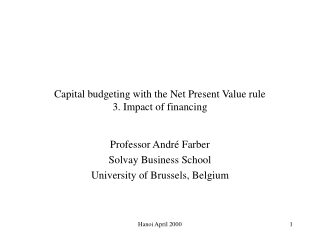# Capital budgeting with the Net Present Value rule 3. Impact of financing - PowerPoint PPT PresentationDownload PresentationCapital budgeting with the Net Present Value rule 3. Impact of financing

Capital budgeting with the Net Present Value rule 3. Impact of financing
Download Presentation## Capital budgeting with the Net Present Value rule 3. Impact of financing

- - - - - - - - - - - - - - - - - - - - - - - - - - - E N D - - - - - - - - - - - - - - - - - - - - - - - - - - -
##### Presentation Transcript

1. Capital budgeting with the Net Present Value rule3. Impact of financing Professor André Farber Solvay Business School University of Brussels, Belgium Hanoi April 2000

2. Capital budgeting for the levered firm • With debt and equity, decision depends on financing mix. • Why? • Tax subsidy to debt • Cost of issuing new securities • Subsidies to debt financing • Cost of financial distress • 3 methods: • Adjusted-Present Value • Flow-to-equity • Weighted-average cost of capital Hanoi April 2000

3. Adjusted-Present-Value • Divide and conquer! • Step 1: Calculate NPV for unlevered project NPV • Step 2: Calculate NPV of financing side NPVF • Step 3: Add up. APV = NPV + NPVF Hanoi April 2000

4. APV - example • Initial investment = 500 • Expected future EBIT = 140 per year for indefinite future • Corporate tax rate = 40% • Cost of capital, all equity r0 = 20% • Unlevered cash flow (UCF) = 140 (1 - 0.40) = 84 • Present value of UCF = 84 / 0.20 = 420 • NPV = -500 + 420 = -80 • Unlevered project would be rejected! Hanoi April 2000

5. APV example: Introducing debt • Now imagine the company borrows 300 • Remaining investment (500 - 300 = 200) financed with equity. • Borrowing rate = 10% • Income statement unlevered levered EBIT 140 140 Interest 0 30 Taxes 56 44 Earnings 84 66 Cash to investors 84 96 Tax saving = 56 - 44 = 12 = 40% x 30 Hanoi April 2000

6. Valuing the Tax Shield • Annual tax shield from debt = TaxRate  Interest rate  Value of debt = TC rB B • Present value (perpetuity) = (TC rB B)/rB =TC B • In our example: PV(TaxShield) = 0.40  300 = 120 Hanoi April 2000

7. APV calculation (finished) • We now have the following for our project: • Net Present Value (all equity) - 80 • Present Value of Tax Shield +120 • Adjusted Present Value + 40 Hanoi April 2000

8. Flow-to-Equity Approach • Valuation of cash flows from the project to the equityholders of levered firm (levered cash flow LCF). • LCF = UCF - (1-TC) x rB x B = UCF - rB x B + TCx rB x B InterestTaxShield • In our example: LCF = 84 - 30 + 12 = 66 Hanoi April 2000

9. Discounting Levered Equity Cash Flows • Because of debt, equity is more risky. Discount rate should take this additional risk into account. • The formula for the discount rate is: rS = r0 + (r0 - rB) x L/(1-L) • with L : debt-to-value ratio • In our example: L = 300 /(420 + 120) = 0.5555 • rS = 0.20 + (0.20 - 0.10) x 0.60 x 1.25 = 27.50% • Present value of levered cash flows = 66/.275 = 240 • NPVfor stockholders = 240 - 200 = 40 … same as APV Hanoi April 2000

10. Weighted-Average-Cost-of-Capital Approach • Discount unlevered cash flow using adjusted cost of capital. • rWACC = rs(1-L) + rB(1-TC) L • (Remember: L = B/V so 1-L = S/V) • In our example: • rWACC = 0.275 x 0.445 + 0.10 x 0.60 x .555 = 15.57% • Net present value = -500 + 84 / 0.1557 = 40 Hanoi April 2000

11. Alternative WACC formulas • Modigliani Miller : rWACC = r0(1-TCL) • perpetuity • debt level constant • Miles-Ezzel: rWACC = r0 - L rB TC(1+r0)/(1+rB) • any set of cash flows • debt ratio constant Bt = L x Vt Hanoi April 2000

12. Comparing APV, FTE and WACC • Which approach is best? • APV • any type of side effect • unbundles present value • use APV when level of debt known • WACC, FTSE • takes into account interest tax shield • use WACC or FTSE when debt ratio constant Hanoi April 2000